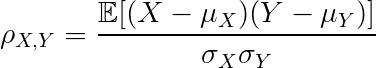## Blog

Home » Practicing Principles » Modern Causal Inference » Augmenting » On media » Causal vs. Statistical Inference

## Causal vs. Statistical Inference

Why is correlation not enough, or is correlation enough? The question bugging the scientific community for a century. A machine learning view on the subject.

Causal inference, or the problem of causality in general, has received a lot of attention in recent years. The question is simple, is correlation enough for inference? I am going to state the following, the more informed uninformed person is going to pose a certain argument that looks like this:

I hate to break it to you if this is your opinion, but no it is not, it is most certainly not. I can see that it is relatively easy to get convinced that it is, but once we start thinking about it a bit we are easily going to come to the realization that it is not. If you are still convinced otherwise after reading this article, please contact me for further discussion because I would be interested in your line of thought.

For illustrating the point that correlation doesn´t necessarily mean causation, let us take a look at the simplest formula of correlation, or rather the famous Pearson correlation coefficient:For those wondering what the Greek letters stand for, μ is the mean of the respective variable and σ is the standard deviation. The E in the numerator denotes expectation, which is effectively a weighted average over different X-Y realizations, where the weights are their probability.

So this correlation coefficient is in the range from -1 to 1, telling us if the variables are negatively or positively correlated. In other words, when one is over its mean and the other one is over or under its mean at the same time, respectively. This correlation coefficient is named after the famous mathematician Karl Pearson, to whom we owe a great deal. People argue that he is the founder of modern statistics, he also introduced the first university statistics department in the world at University College London. Thank you, professor Pearson. But there is one thing he was not really keen on, and that is the argument of causality.

Notice that there is a problem straight away with the correlation formula, which is that there is no sense of direction. Although two variables can be highly correlated with each other, we don’t really know what caused what. To give you an example, take the weather. If it is raining, you most certainly have clouds. Naturally, you ask yourself the question, what caused the rain. Take the correlation between rain and clouds, you notice that there is a positive correlation. Nice, but so what? Can you really say that the clouds caused the rain and not the rain caused the clouds? No, you cannot, not based on this simple correlation coefficient. Perhaps you would notice though one thing, obviously, the clouds appear before the rain. Then you would realize, but wait, if I introduce a temporal aspect to my variables and calculate something as lagged correlation, then I should realize that the clouds cause rain and not the other way around. This is true, but this brings me to my next argument.

## The problem of chocolate addicted Nobel prize winners

There is one famous study that showed that there is a strong correlation between a country’s chocolate consumption and the number of Nobel prize winners coming from this country. So would you say that chocolate consumption causes one’s probability to become a Nobel prize winner to be higher and start consuming chocolate like crazy immediately? I hope not, I suspect that it is reasonable to expect that chocolate does not cause one to be a Nobel prize winner. So let us extract two variables from this statement. B— Being a Nobel prize winner, A— consuming chocolate. The causal diagram for this statement would basically look like this:

The arrow meaning that A causes B. As you can see, this is a very primitive causal diagram. Now we can come to the point, although we have strong correlation between chocolate consumption and Nobel prize winning, we can ask ourselves, is there some other variable, C, such as the country’s wealth that causes both Nobel prize winning and chocolate consumption, or is it the country’s educational system that causes both and so on. Let us imagine, as indeed is the case, that there is a common cause C for both. Then the causal diagram looks like this:

Now we can mention Reichenbach’s common cause principle which states that if variables A and B have a common cause, C, then when we condition on C, the correlation between these variables is wiped out, meaning that the conditional distributions of the random variables conditioning on the common cause become independent. Nice enough. So actually the causal diagram that we should be looking at is the following: